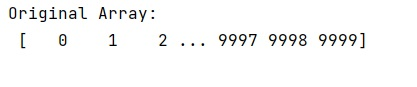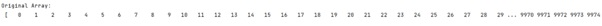# How to set max output width in NumPy?

Learn, how to set max output width in Python NumPy?
Submitted by Pranit Sharma, on February 20, 2023

NumPy is an abbreviated form of Numerical Python. It is used for different types of scientific operations in python. Numpy is a vast library in python which is used for almost every kind of scientific or mathematical operation. It is itself an array which is a collection of various methods and functions for processing the arrays.

## NumPy - Setting max output width

Suppose that we are working on a large array and on printing the array by performing some operation, we face some trouble in seeing the entire array. We need to find a way to set the max output width in numpy.

For this purpose, set_printoptions() method will work. It will take a parameter called linewidth which means only edgeitems and the window's width will determine when newlines/wrapping occurs.

Let us understand with the help of an example,

## Python code to set max output width in NumPy

```# Import numpy
import numpy as np

# Creating a numpy array
arr = np.array([i for i in range(10000)])

# Display original array
print("Original Array:\n",arr,"\n")
```

Output:```np.set_printoptions(edgeitems=30, linewidth=100000,
formatter=dict(float=lambda x: "%.3g" % x))

# Display original array
print("Original Array:\n",arr,"\n")
```

Output: